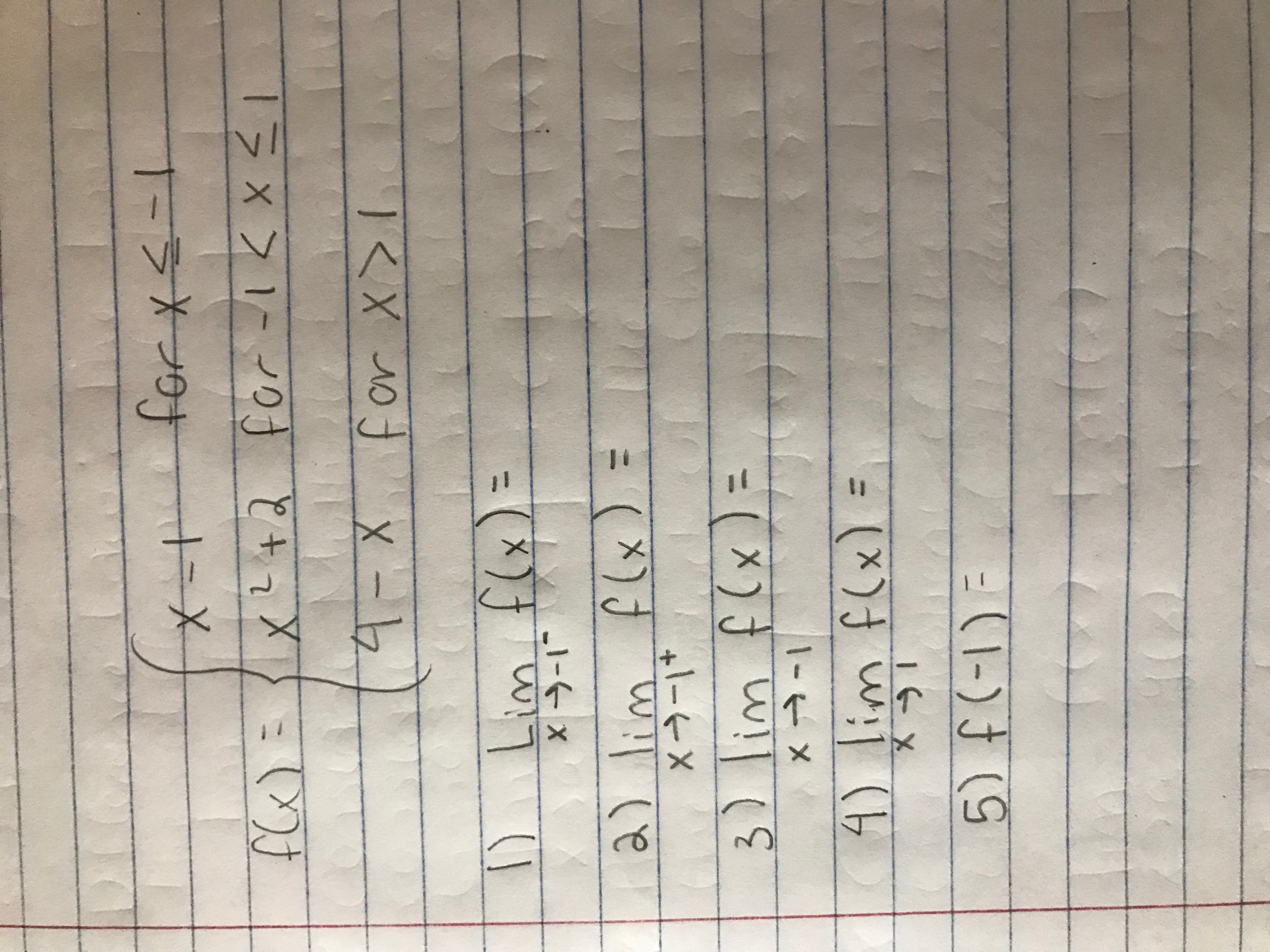# s-for xx-tX-+-1=Lim f(x)XX-1flx) =a) lim3) lim flx)x-14) lim f(x)5) f(-I)E

Question

Sketch the graph of the following function and use it to define the following limits, or specify if a limit does not exist.help_outlineImage Transcriptioncloses- for x x-t X-+ -1 = Lim f(x) X X-1 flx) = a) lim 3) lim flx) x-1 4) lim f(x) 5) f(-I)E fullscreen
check_circleExpert Solution
Step 1

As per norms, the first three questions are answered.

Step 2

The graph of the function (piecewise defined) is sketched. The red points are the actual values at the points x =-1 and x=1

Step 3

1) the required limit is -2, as f(x) approaches x-1 ...

### Want to see the full answer?

See Solution

#### Want to see this answer and more?

Solutions are written by subject experts who are available 24/7. Questions are typically answered within 1 hour*

See Solution
*Response times may vary by subject and question
Tagged in

### Limits